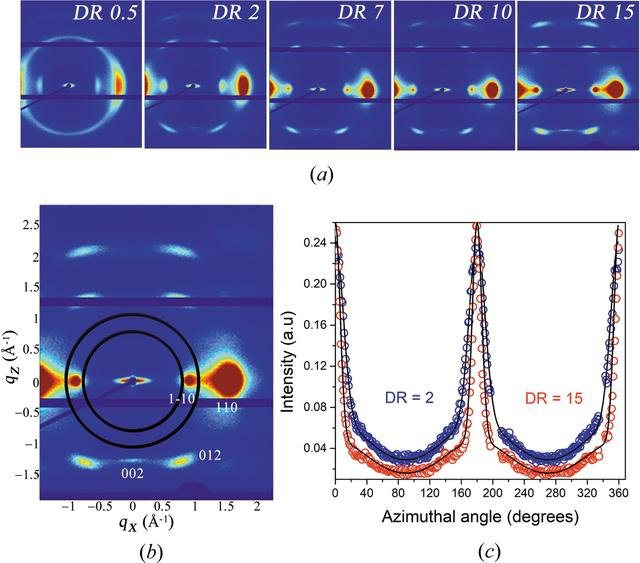disable zoom     view article Figure 1 (a) From left to right, 2D fiber diffraction patterns obtained at different draw ratios DR = 0.5, 2, 7, 10 and 15. (b) A 2D diffraction pattern from the DR = 15 fibers, with assignment of diffraction spots according to cellulose II. The space between the two circles indicates the q band of 0.80–0.95 Å−1, which includes thereflection. (c) Azimuthal plots of the intensity in the q band of 0.80–0.95 Å−1, for two different draw ratios, DR = 2 (blue open circles) and DR = 15 (red open circles). The solid black lines are model fits corresponding to a linear combination of two Gaussian functions, see equation (1).

IUCrJ
Volume 9| Part 4| July 2022| Pages 492-496
ISSN: 2052-2525